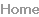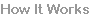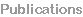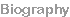The termination of the magnetic field is a critical step in the VPPEM because it produces the angular image, and allows the photoelectrons produced within the magnetic field to be energy analyzed outside the field using a band pass analyzer.
We need to understand how to design the field termination using an
aperture in the ferromagnetic shield.

We explore the effects of field termination using a simple model
system.

For a model system, we choose a central solenoid of sufficient field
strength to give a meaningful result, but not too strong that it would
saturate a single layer ferromagnetic shield.

There are many possible arrangements, and the model system used
here is not intended to be an optimum design for an actual VPPEM.

Two magnetic circuits are modelled to give different field strengths at
the exit aperture.Page 1
Page 2Page 3The first magnetic circuit is shown in Figure 1. This configuration has
a ferromagnetic shield 20.0 cm long, and 7.5 cm in radius, with a 2.5
cm thick 1018 steel shield. The solenoid is 5.0 cm long with a 2.0 cm
inner radius and 3.0 cm outer radius.

The solenoid current produces an on-axis field maximum at the
sample position of 0.5 Tesla. The field near the exit aperture at 7.5
cm is in the region of 0.02 Tesla.Figure 1 Model system of a solenoid in a ferromagnetic shield with an on-axis
aperture at the right. The model is radially symmetric around the axis. The
lines are flux lines and the colors indicate field strength, red highest.
The second magnetic circuit is the same as Figure 1 but with the
shield length increased to 25 cm. The exit aperture is at 12.5 cm from
the center, and the field at the aperture is an order of magnitude lower
at 0.002 Tesla.
Figure 2a shows a plot of Bz from the center of the solenoid at 0.0 cm
to the end of the aperture at 10.0 cm. This plot is for the smallest
aperture of 0.4 mm.

The field decays smoothly from 0.5 Tesla until it reaches a ‘knee’ at
approximately 0.25 Tesla near the aperture at 7.5 cm, and then
decays rapidly to zero.  A comparison of the shape Bz for the three
aperture sizes is shown in Figure 2b.
These two configurations give us a high, and low field termination for comparison. The
other variables used in the calculations are aperture diameters 0.4 mm, 2.0 mm, and
2.0 cm, and electron energies 10.0 eV, and 100.0 eV.

The magnetic field and trajectories are modelled using Field Precision 2D TriComp
suite of programs, and the colored plot of |B| shown in Figure 1 is imaged using 2D
Infolytica MagNet software.

We are interested in the angular magnification, and the relationship between θ and φ
given these thee parameters of terminating field, aperture diameter, and electron energy.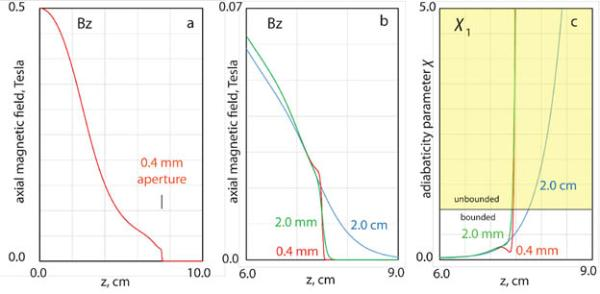Figure 2 shows the shape of the axial field, Bz, for the first magnetic
field circuit, the high exit field configuration.
Figure 2 Comparison of the field shape for three different aperture sizes for the high field model of Figure 1, a)
the axial field for a 0.4 mm aperture from the center of the solenoid at 0.0 cm to the end of the ferromagnetic
shield at 10 cm, b) comparison of the axial field around the aperture position at 7.5 cm for three aperture
sizes, c) comparison of the adiabaticity parameter for three aperture sizes.

Figure 2b shows that as the aperture becomes larger the ‘knee’ of
the field in the region of the aperture at 7.5 cm becomes more
rounded, and the field termination is less abrupt. For the 2.0 cm
aperture, the field is somewhat lower at the aperture.
Figure 3 shows the trajectories for 10.0 eV electrons exiting from the
three apertures for a 1 micron off-axis position at the sample, and the
high field termination.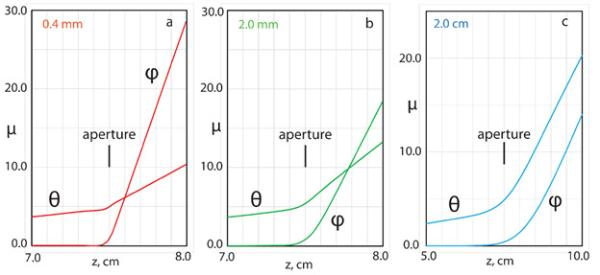Figure 3 Trajectories for 10.0 eV electrons leaving the magnetic field of the high field model of Figure 1, a)
aperture 0.4 mm ID, b) aperture 2.0 mm ID, c) aperture 2.0 cm ID.  The in-plane deflection is φ, and the
transverse out-of-plane deflection is θ. The position of the start of the aperture at 7.5 cm is indicated.Electrons can be accelerated in the strong magnet field without
distorting the image.

VPPEM normally images using very low energy electrons at the
sample, typically around 1.0 eV. A sample bias is used to for
insertion into the CHA input lens. This means that energy of the
electrons at the exit aperture can be easily changed to manipulate
the focal properties of the instrument.

There are some general trends as the aperture size increases,
and the field termination becomes less abrupt.

The radial deflection, Φ, decreases from 5.8x10-3 radians for the
0.4 mm aperture to 1.5x10-3 for the 2.0 cm aperture. At the same
time, the relative sizes of the θ and φ deflection change.

This leads to a change in the out-of-plane image rotation, Ψ, which
changes from 76o for the 0.4 mm aperture to 42o for the 2.0 cm
aperture.We have simulated electron trajectories over a range of electron
energies, however, we only present the results for 10.0 eV and 100.0
eV. All results are for trajectories 1.0 micron off the axis at the center of
the 0.5 Tesla field.

Table 1 summarizes the results for numerical simulations of the
trajectories for the two field strengths, three aperture sizes, and two
energies. These results are discussed below.
Table 1 Results from electron trajectory calculations for two magnetic circuits based on Figure 1.
The nominal high and low field values at the apertures are 0.02 and 0.002 Tesla respectively.Page 4
To understand, and extend the systematics in the results, we
need a guide. For this we can use the adiabaticity parameter χ1
of Kruit and Reed ref 5. The adiabaticity parameter was
developed to find the boundary of useful behavior for magnetic
projection microscopes such as Beamson et al. refs 6,7,
Pianetta et al. refs 8,9, and Kruit and Reed’s magnetic
paralleliser ref 5.  The adiabaticity parameter χ1 is given by:Where the electron energy E is in electron volts, and Bz in Tesla.

The parameter is a factor based on the ratio of change of
magnetic field within one cyclotron orbit. Kruit and Reed
suggested that for values of χ1 less than unity that the electron
trajectories would stay bounded to the magnetic field lines. As the
electrons move into a region with a high χ1, they will leave the
point out.

This formula shows χ1 increases with a decrease in Bz, and an
increase in energy. Also, the sharper the field termination the
larger χ1.
In Figure 2c, χ1 is plotted for 10 eV electrons for the three
aperture sizes in the high field case. For illustration, a sharp
boundary is indicated at χ1 = 1.0.

In figure 4c the 0.4 mm and 2.0 mm apertures have a very sharp
transition from bounded to unbounded. For the 2.0 cm aperture,
the boundary is shifted down the z axis, and the behavior on
exiting the field might be expected to look different.
plane deflection begins just before the aperture. This is at the same point that
value of χ1 goes above unity in Figure 3c. The in-plane deflection at this point
gives a magnification of x4.45 at the aperture implying the magnetic field at this
point is 0.0245 Tesla. This value is near the top of the knee in the plot of Figure
3b for the 0.4 mm aperture.

An estimate of the defection can be made from the vector potential Aexit where
the electrons leave the field lines:
The ratio of these two momenta gives a deflection angle of 5.2x10-3 radians for
10.0 eV electrons. The value measured from the φ trajectory deflection is 5.6
x10-3 radians. This figure is 7% higher than the estimate from the vector
potential calculation. Close inspection of the φ trajectory shows it moves off the
axis before leaving the field lines. This off-axis movement gives an additional
deflection. The trajectories are still complex even for a sharp transition.

From Table 1, we can compare the results for different apertures, energies,
and exit fields.  There are several trends.

First, the deflection angles for large apertures is lower. This trend can also be
observed in Figure 3. For the 0.4 mm aperture, and 10.0 eV electrons in a high
field, Φ the radial deflection is 5.6x10-3 radians. This figure drops to 4.2x10-3
radians for the 2.0 mm aperture, and the angle for the larger 2.0 cm aperture is
significantly lower at 1.5x10-3 radians.

Second, the relative values of the φ and θ contributions change. For the 0.4 mm
aperture, Ψ the rotation of the deflection, is 76o, while for the 2.0 cm aperture it
is 42o. With the point of exit from the field extending out to a weaker field value,
as indicated in Figure 2c, the curvature of the trajectories in the field add to the
in-plane deflection, while the vector potential out-of-plane deflection becomes
much weaker.
We would like to fix where the trajectories leave the magnetic field
because we want to estimate the deflection due to the vector
potential at this point, and compare it to trajectory plots. This is
more straightforward for the smaller 0.4 mm aperture.
energy electrons would be expected to be sharper, and start earlier.  Which is
generally observed. Moreover, the rotation angles tend towards higher angles,
and the relative deflections Φ10/ Φ100 with a factor ten times the energy,
become closer to the square root of ten. These trends are followed for small
apertures. However, Table 1 shows that for the larger 2.0 cm aperture, the
trends are more complex.Page 5
mm aperture is estimated by observing where the φ deflection
leaves the axis. This is much clearer for the small aperture where
deflection is used to give the field, and by calculation the
magnification.

In terms of the electron optical design process, where rules of
towards a solution, the results in Table 1 for the 2.0 cm aperture
are particularly noteworthy. We would naturally expect that
increasing the electron energy by a factor ten would reduce the
radial deflection Φ by a factor 3.12, and reducing the exit field by a
factor ten would produce a corresponding decrease in Φ. This is
clearly not the case. The change in deflection for the high field
case is only 1.64 for a factor ten change in energy, and for 10.0 eV
electrons, the deflection is the same for a factor ten change in
field.

While it is confusing to see that deflections for the 2.0 cm
aperture do not follow the inverse square of the electron energy,
understanding the details make it clearer. The θ deflection is due
to the projection magnification, while the φ deflection is due to the
vector potential.

The projection magnification is the same for all energies, and the
θ deflection will be similar for the similar magnifications. If the
higher energy electron exits the field lines sooner, then it will see
slightly less magnification, but a relatively larger vector potential.
We would then expect that the φ deflection would be larger, and
the Ψ rotation out of plane larger. This is what we see.

For our purposes of instrument design, although the data in
Table 1 is useful, we can only extract rather general rules of
thumb from the trends. We will need to window the actual design
area, and move in from there. Any design will be based on
detailed calculation using trends as a guide.
Page 6

1 R. Browning, Rev. Sci. Instrum. 82, 103703 (2011)

2 R. Browning, Rev. Sci. Instrum. 85, 033705 (2014)

3 R. Browning, J. Elec. Spectros. Relat. Phenom. 195, 125 (2014)

4 R. Browning, Surf. Interface Anal. 47, 63 (2015)

5 P. Kruit, and F. H. Reed, J. Phys. E 16, 313 (1983).

6 G. Beamson, Q. Porter, and D. W. Turner J. Phys. E: Scient. Instrum. 13
64-66 (1980)

7 G. Beamson, Q. Porter, and D. W. Turner, Nature 290, 556 (1981)
.
8 P. Pianetta, I. Lindau, P. L. King, M. Keenlyside, G. Knapp, and R.
Browning, Rev. Sci. Instrum.  60, 1686 (1989).
Conclusion
To design a VPPEM instrument, we need to have an understanding of the
have shown that the vector potential estimate is reliable for a sharp size.

From the complexities of the trends across the three-dimensional
parameter space we have used here, it is clear that most of the design work
for any VPPEM desing requires a full numerical calculation.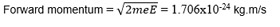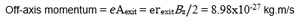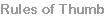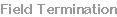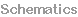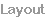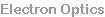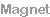Field Termination
These pages cover different aspects designing a VPPEM instrument
 Instrument Design